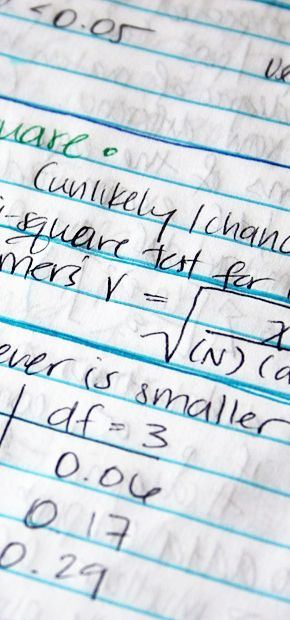+27 83 349 4636

Teaching and Training

Courses taught (Nelson Mandela University)

Introduction to Statistical Inference

Maximum likelihood estimation, Least squares estimation, Sufficient statistics, Confidence intervals, Unbiased estimators, Consistent estimators, Efficient estimators, Fisher information, Statistical hypothesis tests for means, variances and proportions, Neyman Pearson Lemma, Uniform Most Powerful Tests, Analysis of Categorical Data. All practicals conducted using R software.

Data: Acquisition, management, patterns and summary measures. Probability: Basic probability concepts, random variables, expected value. Discrete probability distributions: Bernoulli, Binomial and Poisson. Continuous probability distributions: Uniform, Normal and Exponential. Statistical sampling. Point Estimation and the sampling distribution of the sample mean. Interval estimation of population mean. Statistical test for population mean, proportion and variance. Comparisons of two populations. Simple linear regression analysis. Time series analysis.

Nonparametric Statistical Procedures

Binomial, Quantile, Sign and McNemar Tests, Contingency Tables and χ2-tests, Median, Mann-Whitney, Kruskal Wallis, Siegal-Tukey, Wilcoxon Signed Ranks and Shapiro Wilks Tests, Spearman Rho and Kendall Tau tests of association. All practicals conducted using R software.

Data Skills for Zoologists

Measures of Location and Dispersion, Basic Probability, Normal Distribution, Sampling Distributions, Confidence Intervals, Sample Size, Hypothesis Testing, Nonparametric tests, Analysis of Variance, Regression, Multiple Regression, Correlation, Analysis of Categorical Data, Multivariate Analysis and Data Management. All practicals conducted using R software.Completed Students (Nelson Mandela University)

• Bracken van Niekerk (Masters) - The Application of Hidden Markov Models and their Extensions to Animal Movement Data.
• Bracken van Niekerk (Honours) - The Application of Independent Mixture Models and Hidden Markov Models to Eland Movement Data in Two Regions in the Eastern Cape.
• Lee Watchurst (Honours) - Presence and Movement Modelling of Leopards.
• Jennica Poongavanan (Honours) - A Novel Approach at Uncovering the Latent Movement States and Predicting Kill-Sites of Lions using Hidden Markov Models.
• Motladi Matatiele (Honours) - African Penguin chick growth rate in Algoa Bay across four decades of contrasting environmental conditions.
• Kagiso Besele (Honours) - Risk modelling for white rhino TB associated challenges.

Current Students (Nelson Mandela University)

• Michaela Ritchie (PhD) - Statistical Classification at a Pixel and Sub-Pixel level of Remote Sensing Imagery in the False Bay Region.